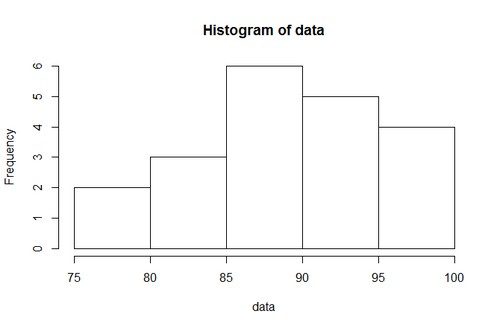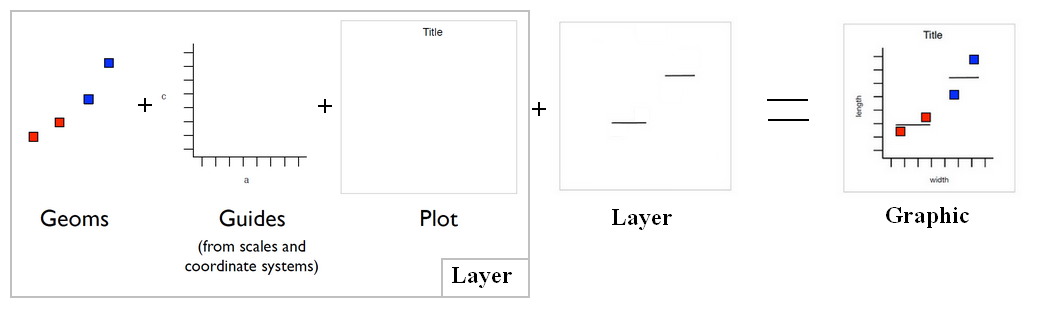# R - Plot (Graphic System)

## Formula

The general form of a formula specifying a plot is

````y ˜ x | g`
```

where:

• y is the coordinate on the vertical axis,
• x is the coordinate on the horizontal axis,
• and g is a grouping factor or expression.

For special cases like the histogram, the vertical axis is pre-specified. In these cases y is omitted.

## Graphic System

The R graphics system is composed of:

• graphics packages;
• graphics systems:
• a graphics engine and devices;
• and graphics device packages

### Device

All graphics output is directed to an active device that will dictate the output format that will be produced.

Only one device can be currently active and receive the graphics output.

``````# Open a device
dev.new()
# Close a device
dev.off()
# List of open devices
dev.list()
# List of the current (ie active) open device
dev.cur()
# Make a device active
dev.set()
# Copies all output from the active device to another device.
dev.copy()
# Make the next or previous device active
dev.next() and dev.prev()
# Close all open devices
graphics.off()```
```

Example in png.

``````png(file="myplot.png")
plot(data)
dev.off()```
```

The user can control the format of the default device using the options() function.

List:

## Syntax

``````plot(reponseVariable~predictorVariable,data.frame)
### Or
plot(data.frame\$predictorVariable,data.frame\$reponseVariable)```
```

See help

````?plot`
```

## Character

Plotting character (pch)

An easy way to see them all:

````plot(1:20,1:20,pch=1:20,cex=2)`
```

## Documentation / Reference

Discover MoreR - Feature Selection - Model selection with Direct validation (Validation Set or Cross validation)

Feature selection through model generation and selection through a direct approach with : validation set and cross validation in R We are picking a subset of the observations and put them...R - Histogram

How to make histogram in R. plot“” You can use the layout function in order to have more than one histogram by page. Example: See : Histogram: Geom: bar Stat: bin Scale: linear...R - Non-linear Effect Analysis

Non-linear Analysis with R. where: the quadratic is indicated by the power 2 (predictor1^2) As power has a meaning in this formula, the identity function (I) is used to protect it....ggplot - Quick Plot (qplot)

Wraps up all the details of ggplot with a familiar syntax borrowed from plot Automatically scales data Can produce any type of plot Facetting and margins Creates objects that can be saved and...